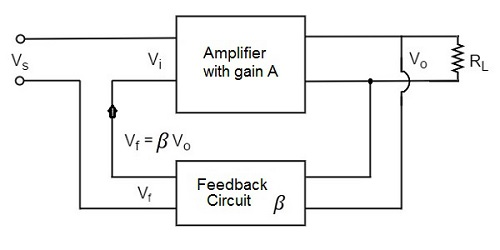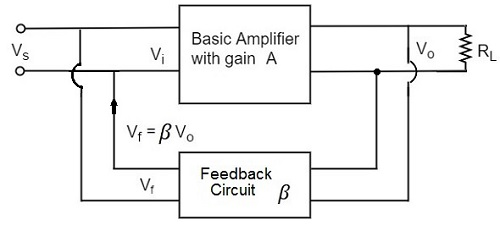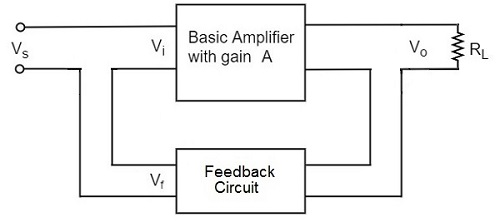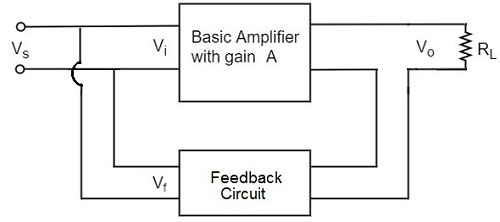# Amplifiers Negative Feedback

Advertisements

Negative feedback in an amplifier is the method of feeding a portion of the amplified output to the input but in opposite phase. The phase opposition occurs as the amplifier provides 180o phase shift whereas the feedback network doesn’t.

While the output energy is being applied to the input, for the voltage energy to be taken as feedback, the output is taken in shunt connection and for the current energy to be taken as feedback, the output is taken in series connection.

There are two main types of negative feedback circuits. They are −

• Negative Voltage Feedback
• Negative Current Feedback

## Negative Voltage Feedback

In this method, the voltage feedback to the input of amplifier is proportional to the output voltage. This is further classified into two types −

• Voltage-series feedback
• Voltage-shunt feedback

## Negative Current Feedback

In this method, the voltage feedback to the input of amplifier is proportional to the output current. This is further classified into two types.

• Current-series feedback
• Current-shunt feedback

Let us have a brief idea on all of them.

## Voltage-Series Feedback

In the voltage series feedback circuit, a fraction of the output voltage is applied in series with the input voltage through the feedback circuit. This is also known as shunt-driven series-fed feedback, i.e., a parallel-series circuit.

The following figure shows the block diagram of voltage series feedback, by which it is evident that the feedback circuit is placed in shunt with the output but in series with the input.As the feedback circuit is connected in shunt with the output, the output impedance is decreased and due to the series connection with the input, the input impedance is increased.

## Voltage-Shunt Feedback

In the voltage shunt feedback circuit, a fraction of the output voltage is applied in parallel with the input voltage through the feedback network. This is also known as shunt-driven shunt-fed feedback i.e., a parallel-parallel proto type.

The below figure shows the block diagram of voltage shunt feedback, by which it is evident that the feedback circuit is placed in shunt with the output and also with the input.As the feedback circuit is connected in shunt with the output and the input as well, both the output impedance and the input impedance are decreased.

## Current-Series Feedback

In the current series feedback circuit, a fraction of the output voltage is applied in series with the input voltage through the feedback circuit. This is also known as series-driven series-fed feedback i.e., a series-series circuit.

The following figure shows the block diagram of current series feedback, by which it is evident that the feedback circuit is placed in series with the output and also with the input.As the feedback circuit is connected in series with the output and the input as well, both the output impedance and the input impedance are increased.

## Current-Shunt Feedback

In the current shunt feedback circuit, a fraction of the output voltage is applied in series with the input voltage through the feedback circuit. This is also known as series-driven shunt-fed feedback i.e., a series-parallel circuit.

The below figure shows the block diagram of current shunt feedback, by which it is evident that the feedback circuit is placed in series with the output but in parallel with the input.As the feedback circuit is connected in series with the output, the output impedance is increased and due to the parallel connection with the input, the input impedance is decreased.

Let us now tabulate the amplifier characteristics that get affected by different types of negative feedbacks.

Characteristics Types of Feedback
Voltage-Series Voltage-Shunt Current-Series Current-Shunt
Voltage Gain Decreases Decreases Decreases Decreases
Bandwidth Increases Increases Increases Increases
Input resistance Increases Decreases Increases Decreases
Output resistance Decreases Decreases Increases Increases
Harmonic distortion Decreases Decreases Decreases Decreases
Noise Decreases Decreases Decreases Decreases
Advertisements